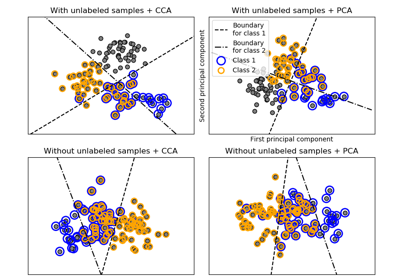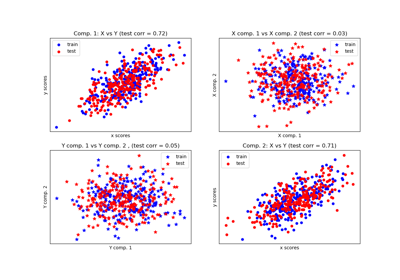# sklearn.cross_decomposition.CCA¶

class sklearn.cross_decomposition.CCA(n_components=2, scale=True, max_iter=500, tol=1e-06, copy=True)[source]

CCA Canonical Correlation Analysis.

CCA inherits from PLS with mode=”B” and deflation_mode=”canonical”.

Read more in the User Guide.

Parameters: n_components : int, (default 2). number of components to keep. scale : boolean, (default True) whether to scale the data? max_iter : an integer, (default 500) the maximum number of iterations of the NIPALS inner loop tol : non-negative real, default 1e-06. the tolerance used in the iterative algorithm copy : boolean Whether the deflation be done on a copy. Let the default value to True unless you don’t care about side effects x_weights_ : array, [p, n_components] X block weights vectors. y_weights_ : array, [q, n_components] Y block weights vectors. x_loadings_ : array, [p, n_components] X block loadings vectors. y_loadings_ : array, [q, n_components] Y block loadings vectors. x_scores_ : array, [n_samples, n_components] X scores. y_scores_ : array, [n_samples, n_components] Y scores. x_rotations_ : array, [p, n_components] X block to latents rotations. y_rotations_ : array, [q, n_components] Y block to latents rotations. n_iter_ : array-like Number of iterations of the NIPALS inner loop for each component.

Notes

For each component k, find the weights u, v that maximizes max corr(Xk u, Yk v), such that |u| = |v| = 1

Note that it maximizes only the correlations between the scores.

The residual matrix of X (Xk+1) block is obtained by the deflation on the current X score: x_score.

The residual matrix of Y (Yk+1) block is obtained by deflation on the current Y score.

References

Jacob A. Wegelin. A survey of Partial Least Squares (PLS) methods, with emphasis on the two-block case. Technical Report 371, Department of Statistics, University of Washington, Seattle, 2000.

In french but still a reference: Tenenhaus, M. (1998). La regression PLS: theorie et pratique. Paris: Editions Technic.

Examples

>>> from sklearn.cross_decomposition import CCA
>>> X = [[0., 0., 1.], [1.,0.,0.], [2.,2.,2.], [3.,5.,4.]]
>>> Y = [[0.1, -0.2], [0.9, 1.1], [6.2, 5.9], [11.9, 12.3]]
>>> cca = CCA(n_components=1)
>>> cca.fit(X, Y)
...
CCA(copy=True, max_iter=500, n_components=1, scale=True, tol=1e-06)
>>> X_c, Y_c = cca.transform(X, Y)


Methods

 fit(X, Y) Fit model to data. fit_transform(X[, y]) Learn and apply the dimension reduction on the train data. get_params([deep]) Get parameters for this estimator. predict(X[, copy]) Apply the dimension reduction learned on the train data. score(X, y[, sample_weight]) Returns the coefficient of determination R^2 of the prediction. set_params(**params) Set the parameters of this estimator. transform(X[, Y, copy]) Apply the dimension reduction learned on the train data.
__init__(n_components=2, scale=True, max_iter=500, tol=1e-06, copy=True)[source]
fit(X, Y)[source]

Fit model to data.

Parameters: X : array-like, shape = [n_samples, n_features] Training vectors, where n_samples is the number of samples and n_features is the number of predictors. Y : array-like, shape = [n_samples, n_targets] Target vectors, where n_samples is the number of samples and n_targets is the number of response variables.
fit_transform(X, y=None)[source]

Learn and apply the dimension reduction on the train data.

Parameters: X : array-like, shape = [n_samples, n_features] Training vectors, where n_samples is the number of samples and n_features is the number of predictors. y : array-like, shape = [n_samples, n_targets] Target vectors, where n_samples is the number of samples and n_targets is the number of response variables. x_scores if Y is not given, (x_scores, y_scores) otherwise.
get_params(deep=True)[source]

Get parameters for this estimator.

Parameters: deep : boolean, optional If True, will return the parameters for this estimator and contained subobjects that are estimators. params : mapping of string to any Parameter names mapped to their values.
predict(X, copy=True)[source]

Apply the dimension reduction learned on the train data.

Parameters: X : array-like, shape = [n_samples, n_features] Training vectors, where n_samples is the number of samples and n_features is the number of predictors. copy : boolean, default True Whether to copy X and Y, or perform in-place normalization.

Notes

This call requires the estimation of a p x q matrix, which may be an issue in high dimensional space.

score(X, y, sample_weight=None)[source]

Returns the coefficient of determination R^2 of the prediction.

The coefficient R^2 is defined as (1 - u/v), where u is the residual sum of squares ((y_true - y_pred) ** 2).sum() and v is the total sum of squares ((y_true - y_true.mean()) ** 2).sum(). The best possible score is 1.0 and it can be negative (because the model can be arbitrarily worse). A constant model that always predicts the expected value of y, disregarding the input features, would get a R^2 score of 0.0.

Parameters: X : array-like, shape = (n_samples, n_features) Test samples. For some estimators this may be a precomputed kernel matrix instead, shape = (n_samples, n_samples_fitted], where n_samples_fitted is the number of samples used in the fitting for the estimator. y : array-like, shape = (n_samples) or (n_samples, n_outputs) True values for X. sample_weight : array-like, shape = [n_samples], optional Sample weights. score : float R^2 of self.predict(X) wrt. y.
set_params(**params)[source]

Set the parameters of this estimator.

The method works on simple estimators as well as on nested objects (such as pipelines). The latter have parameters of the form <component>__<parameter> so that it’s possible to update each component of a nested object.

Returns: self
transform(X, Y=None, copy=True)[source]

Apply the dimension reduction learned on the train data.

Parameters: X : array-like, shape = [n_samples, n_features] Training vectors, where n_samples is the number of samples and n_features is the number of predictors. Y : array-like, shape = [n_samples, n_targets] Target vectors, where n_samples is the number of samples and n_targets is the number of response variables. copy : boolean, default True Whether to copy X and Y, or perform in-place normalization. x_scores if Y is not given, (x_scores, y_scores) otherwise.

## Examples using sklearn.cross_decomposition.CCA¶Multilabel classificationCompare cross decomposition methods